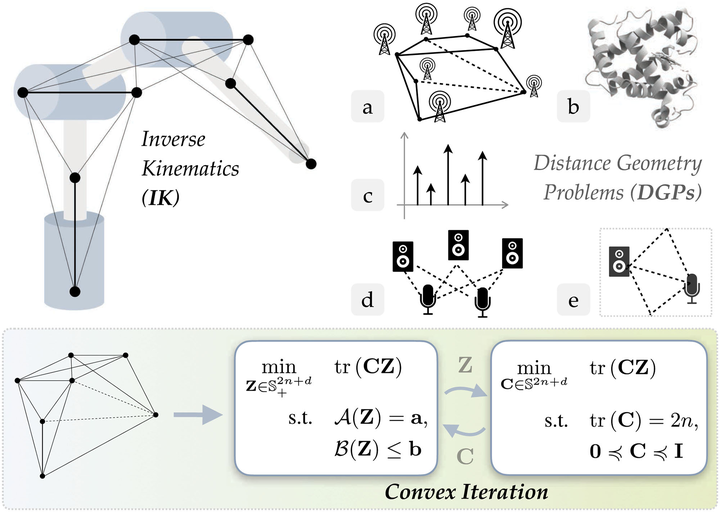# Convex Iteration for Distance-Geometric Inverse Kinematics### Abstract

Inverse kinematics (IK) is the problem of finding robot joint configurations that satisfy constraints on the position or pose of one or more end-effectors. For robots with redundant degrees of freedom, there is often an infinite, nonconvex set of solutions. The IK problem is further complicated when collision avoidance constraints are imposed by obstacles in the workspace. In general, closed-form expressions yielding feasible configurations do not exist, motivating the use of numerical solution methods. However, these approaches rely on local optimization of nonconvex problems, often requiring an accurate initialization or numerous re-initializations to converge to a valid solution. In this work, we first formulate inverse kinematics with complex workspace constraints as a convex feasibility problem whose low-rank feasible points provide exact IK solutions. We then present CIDGIK (Convex Iteration for Distance-Geometric Inverse Kinematics), an algorithm that solves this feasibility problem with a sequence of semidefinite programs whose objectives are designed to encourage low-rank minimizers. Our problem formulation elegantly unifies the configuration space and workspace constraints of a robot: intrinsic robot geometry and obstacle avoidance are both expressed as simple linear matrix equations and inequalities. Our experimental results for a variety of popular manipulator models demonstrate faster and more accurate convergence than a conventional nonlinear optimization-based approach, especially in environments with many obstacles.

Type
Publication
IEEE Robotics and Automation Letters